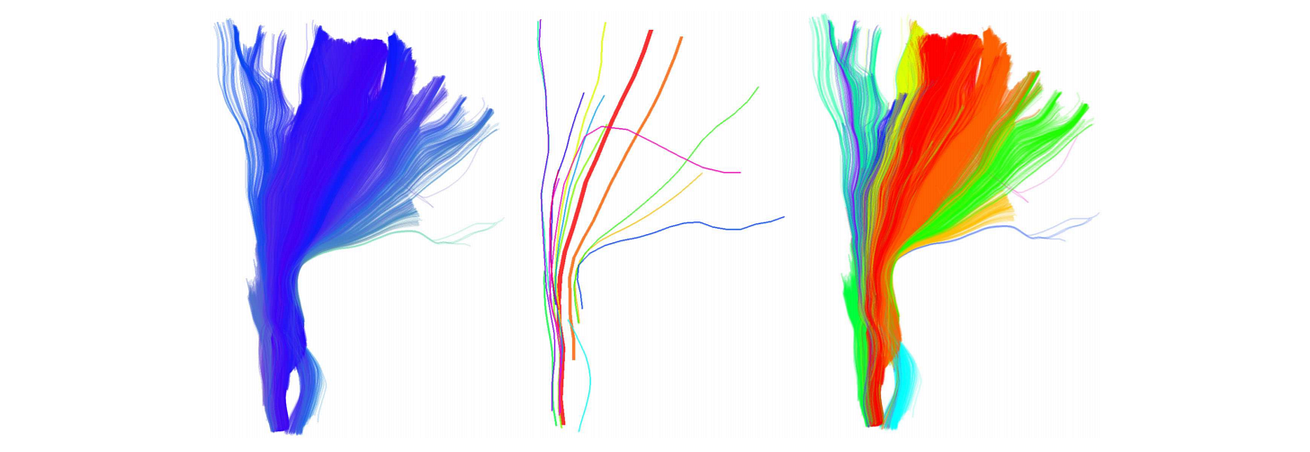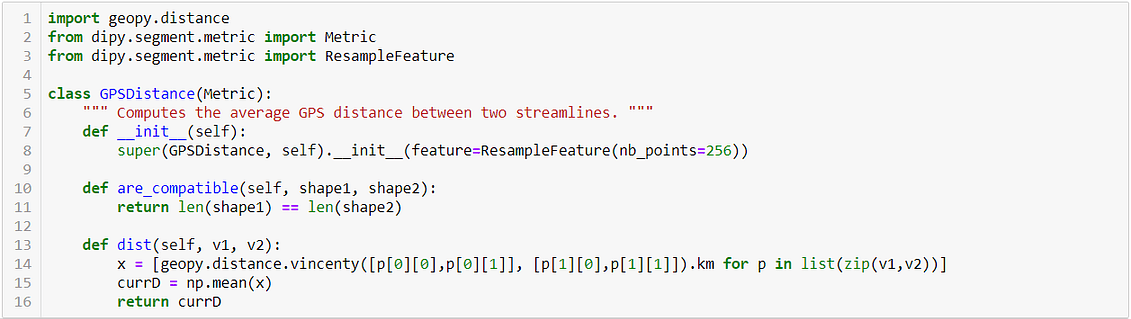written by Claudio Stamile on Dec 7, 2018

GPS trajectories clustering is a common analysis to perform when we want to exploit GPS data generated by personal devices like smartphones or smartwatches.

In this article we will describe a fast and easy way to perform GPS trajectories clustering in Python. Main goal here is to create clusters containing “similar” trajectories. For instance, we want to put in the same cluster the path we follow each day from work to home.

This article is divided in two main parts. In the first, we will describe the clustering algorithm. In the second, we will show how to use and customize the algorithm in Python.

Neuroimage Algorithms and GPS Trajectories Clustering

Instead using classical clustering algorithms like K-Means or DBSCAN, in this article we use a clustering algorithm used in neuromaging.

QuickBundles (QB) is a simple compact clustering algorithm used in magnetic resonance imaging to cluster white matter fibers obtained from the application of tractography algorithms.

Just by looking at the following image, we can see how the white matter fibers in our brain look like GPS trajectories.Example of white matter fibers obtained after application of tractography algorithm. Image from Garyfallidis et al. Frontiers In Neuroscience, vol 6, 2016.

The main idea is to treat each GPS trajectory as a white matter fiber and then merge “similar” trajectories in the same cluster. In the rest of this article we will assume GPS Trajectory = White Matter Fiber.

With this assumption we can then use the description of the algorithm available in the original paper:

The algorithm proceeds as follows. At any one step in the algorithm we have clusters. Select the first streamline s1 and place it in the first cluster c1 ←({1}, s1, 1); M = 1 at this point. For each remaining streamline in turn i = 2, . . ., N:

(i) calculate the distance between streamline si and the centroid streamlines ve of all the current clusters ce e = 1, . . ., M, where v is defined on the fly as v = h/n;

(ii) if any of the distance values me are smaller than a clustering threshold θ, add streamline i to the cluster e with the minimum value for me ce = (I, h, n), and update ce ←(append(I, i), h + s, n + 1); otherwise create a new cluster cM+1 ←([i], si , 1), M ←M + 1. (Page 5 — Garyfallidis et al. Frontiers In Neuroscience, vol 6, 2016)

In the following image we show an example how the algorithm merges the different streamlines in common centroids according to a given threshold value.QuickBundle centroids with different thresholds. Image from Garyfallidis et al. Frontiers In Neuroscience, vol 6, 2016.

The threshold value is THE parameter to select in order to tune the behaviour of the clustering algorithm. If you want the “big” trajectories you can set high values for the threshold. Otherwise, if you want small clusters you need low values.

The authors of the paper provided a Python implementaton of the proposed method. The algorithm is available as part of dipy library while the documentation of the algorithm is available here.

GPS Trajectory Clustering

The dataset we used comes from the GeoLife GPS Trajectories Dataset released by Microsoft Research Asia and available here. A nice overview of the dataset, with examples describing how to open and use it, is available here.

Before starting to perform clustering, let’s plot all the trajectories on google maps using gmplot.

We can now start by defining the distance function between two GPS trajectories (streamlines). Instead using classic Euclidean distance, available in QuickBundle, we will use the GPS distance defined in the GeoPy library.Code to compute the average pointwise distance in GPS trajectories

We computed the average pointwise GPS distance between two trajectories. This way to compute the distance can be used if and only if the two trajectories have the same number of points, this is why we resampled all the trajectories using the ResampleFeature class.

Once the distance between two trajectories is defined, we can run the QuickBundle clustering algorithm.Code to run the trajectory clustering

We can then plot the trajectories contained in the different clusters on google maps using, as before, gmplot.

Here the result in my_map.html plot for different clusters

Conclusions

In this article we described a simple and fast way to perform trajectories clustering of GPS data. The goal was achieved using QuickBundles, a clustering algorithm applied in neuroimaging.

Main limitation of this algorithm is related to the tuning of the threshold parameters. But, as everything in data analysis, this parameter needs to be selected according to the type of clusters you want.

Collected at:  https://medium.com/isiway-tech/gps-trajectories-clustering-in-python-2f5874204a53

2 thoughts on “GPS Trajectories Clustering in Python”

1.oprol evorter says:

Heya i am for the first time here. I found this board and I find It really useful & it helped me out much. I hope to give something back and aid others like you helped me.

2.ปั้มไลค์ says:

Like!! Thank you for publishing this awesome article.